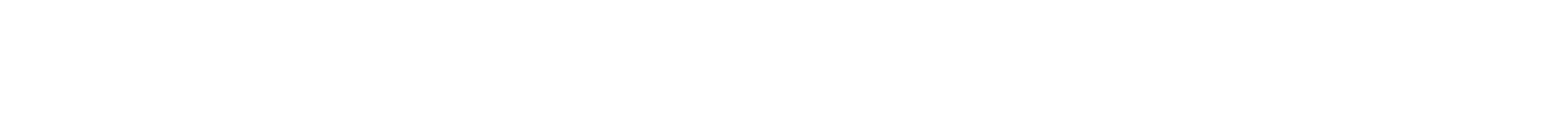# Problem Motivation, Linear Algebra, and Visualization

🎙️ Alfredo Canziani

## Resources

Please follow Alfredo Canziani on Twitter @alfcnz. Videos and textbooks with relevant details on linear algebra and singular value decomposition (SVD) can be found by searching Alfredo’s Twitter, for example type linear algebra (from:alfcnz) in the search box.

## Neural Nets: Rotation and Squashing

A traditional neural network is an alternating collection of two blocks - the linear blocks and the non-linear blocks. Given below is a block diagram of a traditional neural network.Figure 1: Block Diagram of a Traditional Neural Network

The linear blocks (Rotations, for simplicity) are given by:

$\vect{s}_{k+1} = \mW_k z_k$

And the non-linear blocks (Squashing functions for intuitive understanding) are given by:

$\vect{z}_k = h(\vect{s}_k)$

In the above diagram and equations, $$\vx \in \mathbb{R}^n$$ represents the input vector. $$\mW_k \in \mathbb{R}^{n_{k} \times n_{k-1}}$$ represents the matrix of an affine transformation corresponding to the $$k^{\text{th}}$$ block and is described below in further detail. The function $h$ is called the activation function and this function forms the non-linear block of the neural network. Sigmoid, ReLu and tanh are some of the common activation functions and we will look at them in the later parts of this section. After alternate applications of linear and non-linear blocks, the above network produces an output vector $$\vect{s}_k \in \mathbb{R}^{n_{k-1}}$$.

Let us first have a look at the linear block to gain some intuition on affine transformations. As a motivating example, let us consider image classification. Suppose we take a picture with a 1 megapixel camera. This image will have about 1,000 pixels vertically and 1,000 pixels horizontally, and each pixel will have three colour dimensions for red, green, and blue (RGB). Each particular image can then be considered as one point in a 3 million-dimensional space. With such massive dimensionality, many interesting images we might want to classify – such as a dog vs. a cat – will essentially be in the same region of the space.

In order to effectively separate these images, we consider ways of transforming the data in order to move the points. Recall that in 2-D space, a linear transformation is the same as matrix multiplication. For example, the following are transformations, which can be obtained by changing matrix characterictics:

• Rotation (when the matrix is orthonormal).
• Scaling (when the matrix is diagonal).
• Reflection (when the determinant is negative).
• Shearing.
• Translation.

Note that translation alone is not linear since 0 will not always be mapped to 0, but it is an affine transformation. Returning to our image example, we can transform the data points by translating such that the points are clustered around 0 and scaling with a diagonal matrix such that we “zoom in” to that region. Finally, we can do classification by finding lines across the space which separate the different points into their respective classes. In other words, the idea is to use linear and nonlinear transformations to map the points into a space such that they are linearly separable. This idea will be made more concrete in the following sections.

In the next part, we visualize how a neural network separates points and a few linear and non-linear transformations. This can be accessed here.

📝 Rajashekar Vasantha
04 Feb 2021